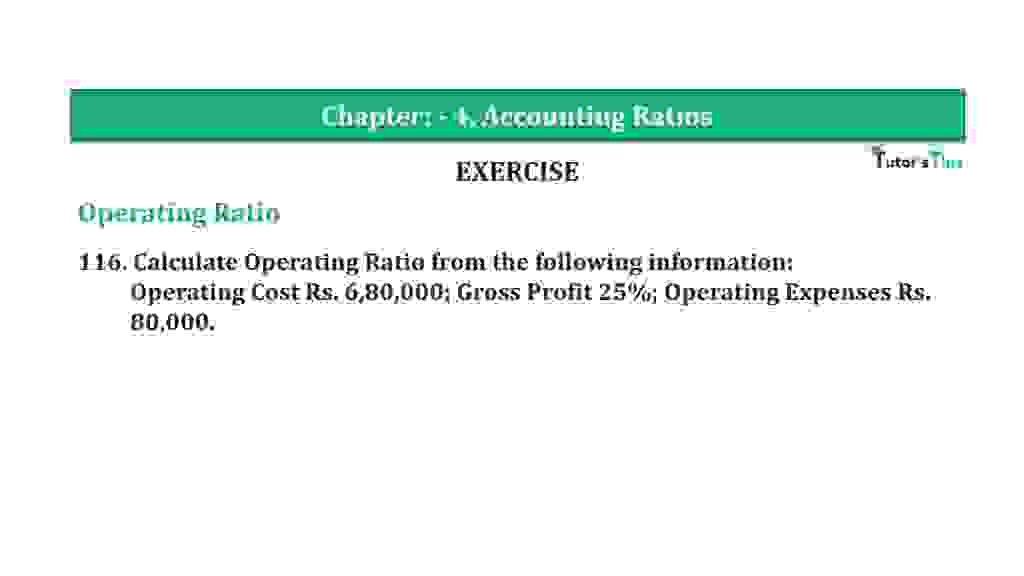# Question 116 Chapter 4 of +2-B – T.S. Grewal 12 ClassQuestion 116 Chapter 4 of +2-B

Operating Ratio

116. Calculate Operating Ratio from the following in formation:
Operating Cost Rs. 6,80,000; Gross Profit 25%; Operating Expenses Rs.
80,000.

### The solution of Question 116 Chapter 4 of +2-B: –

 Operating Cost = Cost of Revenue from Operations + Operating Expenses Rs. 6,80,000 = Cost of Revenue from Operations + Rs. 80,000 = Rs. 6,80,000 – Rs. 80,000 = Rs. 6,00,000
 Gross Profit = 1 X 100 3 = Rs.2,00,000
 Operating Ratio = Gross Profit X 100 Net Sales
 25% = Rs. 2,00,000 X 100 Net Sales
 Net Sales = Rs. 2,00,000 X 100 25% = 8,00,000
 Operating Ratio = Gross Profit X 100 Net Sales
 Net Sales = Rs. 6,80,000 X 100 Rs. 8,00,000 = 85%

Balance Sheet: Meaning, Format & Examples

Thanks, Please Like and share with your friends

Comment if you have any question.

Also, Check out the solved question of previous Chapters: –

### T.S. Grewal’s Double Entry Book Keeping (Vol. II: Accounting for Companies)T.S. Grewal’s Analysis of Financial Statements